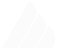# Verly Range Slider

While I was building my physics engine Verly.js I was thinking of making something that would showcase the engine's potential to do some exciting stuff, so I created this just as an experiment.

## How It Works?

It works by using Verly.js's `verly.createRope(x, y, segments, gap, pin)` function which takes arguments - `x` and `y` for location of the rope, `segments` which determines the dots count in the rope (more dots, more fluid rope), `gap` which specifies the distance between each dot (more gap, longer rope), and lastly `pin` which just pins the defined point.

I created a `verly instance` for every input slider present on the screen.

``````let DOMSlider = document.getElementById(id);
let canvas = document.createElement('canvas');
let ctx = canvas.getContext('2d');

// canvas width is equal to the slider's width
let width = DOMSlider.scrollWidth;
let height = width / 2;
canvas.width = width;
canvas.height = height;
canvas.style.pointerEvents = 'none';

// offsetting the position so its lines up
canvas.style.transform = 'translate(0, -15px)';
DOMSlider.parentElement.appendChild(canvas);

// -- then initialized verly
// new Verly(iteration, canvas, ctx)
let verly = new Verly(50, canvas, ctx);// added some gravity
const gravity = new Vector(0, 0.3);``````

after that, I created the rope with

``````// width / 20 to adjust the segments depending on the screen size
let rope = verly.createRope(0, 0, width / 20, 17, 0);let lastIndex = rope.points.length - 1;

rope.setGravity(gravity);
// i pinned the last dot of the rope
rope.pin(lastIndex);``````

#### Getting the slider's position and applying it to the rope

now the actual part where I fix the last dot's position with the slider's thumb. To do this, We will take the slider's `value` and normalize it, so it's between 0 and 1 then multiply the value with the width to get the slider's thumb position.

(yeah i did not bother to use shadowDOM because of browser compatibility and vendor issues)

``````function setRopePosition() {
// get the normalized value of the slider
let ratio = (DOMSlider.value - DOMSlider.min) / (DOMSlider.max - DOMSlider.min);  // then just apply it to the last dot of the rope
rope.points[rope.points.length - 1].pos.x = (ratio * width);
}
// and use event listener

Okay, this works! But I noticed something weird behavior with this.

The problem was, for some reason (probably floating point precision) the slider's position was offsetting a bit while moving towards the edge, so I had to think some ways to prevent this. then I ended up with this solution (yeah crazy but works)

``````function setRopePosition() {
let ratio = (DOMSlider.value - DOMSlider.min) / (DOMSlider.max - DOMSlider.min);

// floating point correction  if (ratio < 0.5) ratio += 0.01;  if (ratio < 0.3) ratio += 0.01;  if (ratio > 0.6) ratio -= 0.01;  if (ratio > 0.8) ratio -= 0.02;
rope.points[rope.points.length - 1].pos.x = (ratio * width);
}``````

nice!

now we can render the scene and update the physics.

``````function animate() {
ctx.clearRect(0, 0, width, height);

verly.update();
// rendering the rope
rope.renderSticks();

requestAnimationFrame(animate);
}
animate();``````

and we are done!

hopefully, you enjoyed playing with this project, I know because I did. you can give the project a star on GitHub if you liked it, have a beautiful day (or night)Made with love and gatsby by anuraghazra